# Vector Algebra class 12 Notes Mathematics

Vector Algebra class 12 Notes Mathematics in PDF are available for free download in myCBSEguide mobile app. The best app for CBSE students now provides Vector Algebra class 12 Notes latest chapter wise notes for quick preparation of CBSE board exams and school-based annual examinations. Class 12 Mathematics notes on chapter 10 Vector Algebra are also available for download in CBSE Guide website.

## Vector Algebra Class 12 Notes Mathematics

Download CBSE class 12th revision notes for chapter 10 Vector Algebra in PDF format for free. Download revision notes for Vector Algebra class 12 Notes and score high in exams. These are the Vector Algebra class 12 Notes prepared by team of expert teachers. The revision notes help you revise the whole chapter 10 in minutes. Revision notes in exam days is one of the best tips recommended by teachers during exam days.

## CBSE Class 12 Mathematics Revision Notes Chapter 10 Vector Algebra

Vector: A quantity that has magnitude as well as direction is called vector.

• Zero Vector: A vector whose intial and terminal point coincide is called a zero vector or a null vector. It is denoted as .
• Co-initial vectors: Two or more vectors having the same initial points are called co-initial vectors.
• Collinear vectors: Two or more vectors are said to be collinear if they are parallel to the same line, irrespective of their magnitudes and directions.
• Equal vectors: Two vectors are said to be equal, if they have the same magnitude and direction regardless of the position of their initial points.
• Negative of a vector: A vector whose magnitude is the same as that of a given vector, but direction is opposite to that of it, is called negative of the given vector.
• Position vector of a point P (x, y) is given as   and its magnitude by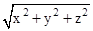• The scalar components of a vector are its direction ratios, and represent its projections along the respective axes.
• The magnitude (r), direction ratios (a, b, c) and direction cosines (l, m, n) of any vector are related as: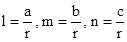• The vector sum of the three sides of a triangle taken in order is
• The vector sum of two conidial vectors is given by the diagonal of the parallelogram whose adjacent sides are the given vectors.
• The multiplication of a given vector by a scalar λ, changes the magnitude of the vector by the multiple |λ|, and keeps the direction same (or makes it opposite) according as the value of λ is positive (or negative).
• For a given vector , the vector gives the unit vector in the direction of
• The position vector of a point R dividing a line segment joining the points P and Q whose position vectors are   and respectively, in the ratio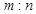(i)  internally, is given by
(ii)  externally, is given by
• The scalar product of two given vectors  and  having angle θ between them is defined as
Also, when is given, the angle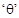between the vectors  and  may be determined by
• If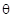is the angle between two vector   and ,  then their cross product is given as
where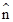is a unit vector perpendicular to the plane containing  and . Such that  form right handed system of coordinate axes.
• If we have two vectors   and  given in component form as   and    and  be  any scalar, then,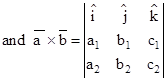Parallelogram Law of vector addition: If two vectors  and  are represented by adjacent sides of a parallelogram in magnitude and direction, then their sum  is represented in magnitude and direction by the diagonal of the parallelogram through their common initial point. This is known as Parallelogram Law of vector addition.

## CBSE Class 12 Revision Notes and Key Points

Vector Algebra class 12 Notes Mathematics. CBSE quick revision note for class-12 Chemistry Physics Math’s, Biology and other subject are very helpful to revise the whole syllabus during exam days. The revision notes covers all important formulas and concepts given in the chapter. Even if you wish to have an overview of a chapter, quick revision notes are here to do if for you. These notes will certainly save your time during stressful exam days.

To download Vector Algebra class 12 Notes Mathematics, sample paper for class 12 Physics, Chemistry, Biology, History, Political Science, Economics, Geography, Computer Science, Home Science, Accountancy, Business Studies and Home Science; do check myCBSEguide app or website. myCBSEguide provides sample papers with solution, test papers for chapter-wise practice, NCERT Vector Algebra, NCERT Exemplar Vector Algebra, quick revision notes for ready reference, CBSE guess papers and CBSE important question papers. Sample Paper all are made available through the best app for CBSE students and myCBSEguide website.Test Generator

Create question paper PDF and online tests with your own name & logo in minutes.myCBSEguide

Question Bank, Mock Tests, Exam Papers, NCERT Solutions, Sample Papers, Notes